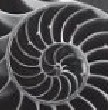Teaching Physics with the Physics Suite Edward F. Redish

Byting the disk

A floppy disk for a computer stores information by magnetizing small regions of the disk. For a typical floppy disk, estimate the area of the disk that corresponds to a single bit of information. (Remember: the storage capacity of a disk is usually given in bytes where 1 byte = 8 bits.)

Solution

Using the first digit of my thumb to measure, I find that a typical floppy disk is about 2.5 inches on a side. This is about (2.5 in) x (2.54 cm/in) or about 6 cm. I know that there is a circular disk inside so it has a radius of about 3 cm. This implies an area of πR2 = about 3 x 3 cm x 3 cm or about 30 cm2. The floppies I use hold 1.44 MB of information. This is 1.44 x 106 bytes = 1.44 x 106 bytes x (8 bits/byte) = about 107 bits. These bits are distributed over an area of 30 cm2 (= 30 x 10-4 m2 = 3 x 10-3 m2) so, assuming that each bit has its own space and there is no overlap, each bit must occupy a space of (3 x 10-3 m2) / (107 bits) = 3 x 10-10 m2. If we think of this as a tiny square, it has dimensions Sqrt(3) x 10-5 m or about 15 micrometers on a side.